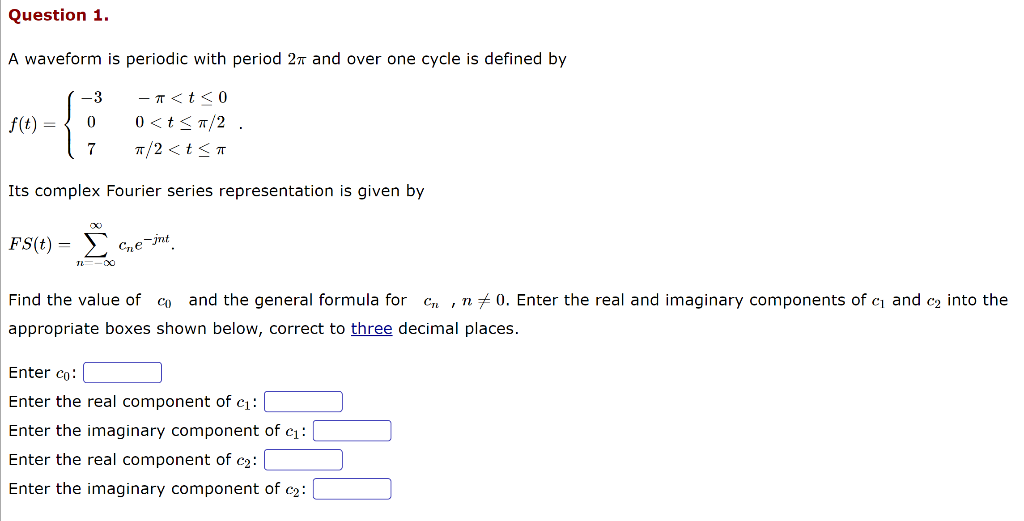Home / Expert Answers / Other Math / question-1-a-waveform-is-periodic-with-period-2-pi-and-over-one-cycle-is-defined-by-f-t-pa574

# (Solved): Question 1. A waveform is periodic with period $$2 \pi$$ and over one cycle is defined by \[ f(t ...Question 1. A waveform is periodic with period $$2 \pi$$ and over one cycle is defined by \[ f(t)=\left\{\begin{array}{cl} -3 & -\pi

We have an Answer from Expert